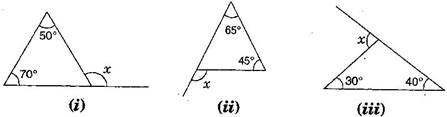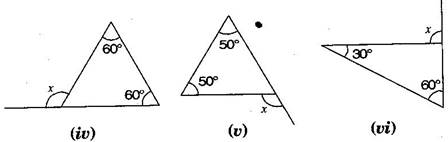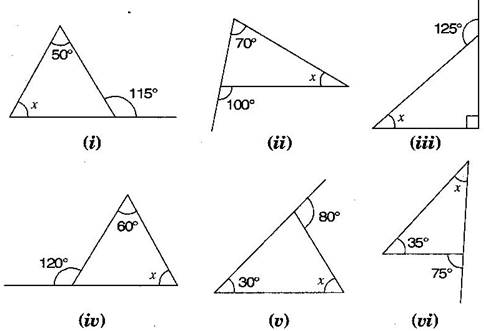### The Triangle and its Properties - Solutions 2

CBSE Class –VII Mathematics
NCERT Solutions
Chapter 6 The Triangle and its Properties
(Ex. 6.2)

Question 1. Find the value of the unknown exterior angle $x$ in the following diagrams:Answer: Since, Exterior angle = Sum of opposite interior angles, therefore
1. $x={50}^{\circ }+{70}^{\circ }={120}^{\circ }$
2. $x={65}^{\circ }+{45}^{\circ }={110}^{\circ }$
3. $x={30}^{\circ }+{40}^{\circ }={70}^{\circ }$
4. $x={60}^{\circ }+{60}^{\circ }={120}^{\circ }$
5. $x={50}^{\circ }+{50}^{\circ }={100}^{\circ }$
6. $x={60}^{\circ }+{30}^{\circ }={90}^{\circ }$
Question 2. Find the value of the unknown interior angle $x$ in the following figures:Answer: Since, Exterior angle = Sum of  opposite interior angles, therefore
1. $x+{50}^{\circ }={115}^{\circ }$ $⇒$ $x={115}^{\circ }-{50}^{\circ }={65}^{\circ }$
2. ${70}^{\circ }+x={100}^{\circ }$ $⇒$ $x={100}^{\circ }-{70}^{\circ }={30}^{\circ }$
3. $x+{90}^{\circ }={125}^{\circ }$ $⇒$ $x={125}^{\circ }-{90}^{\circ }={35}^{\circ }$
4. ${60}^{\circ }+x={120}^{\circ }$ $⇒$ $x={120}^{\circ }-{60}^{\circ }={60}^{\circ }$
5. ${30}^{\circ }+x={80}^{\circ }$ $⇒$ $x={80}^{\circ }-{30}^{\circ }={50}^{\circ }$
6. $x+{35}^{\circ }={75}^{\circ }$ $⇒$